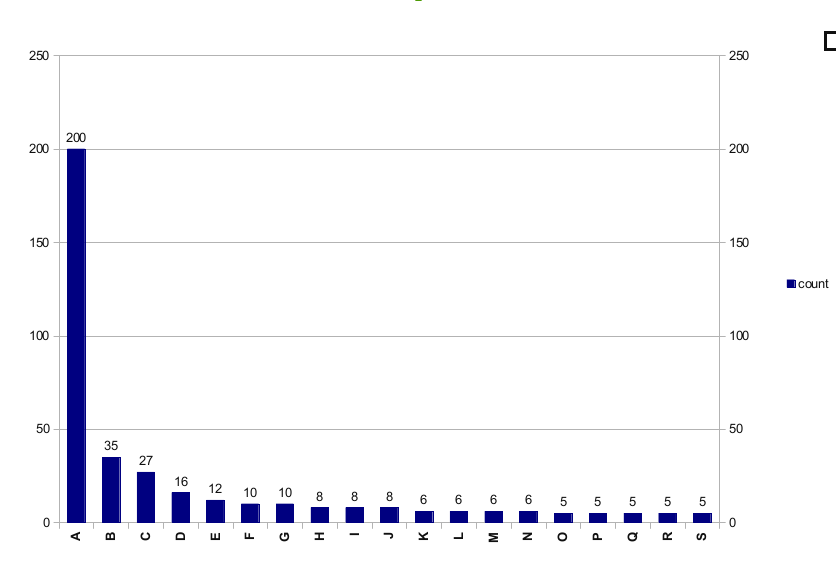# How to make a colored bar chart with named items and values shown above each bar?

Hi,
I would like to create a bar chart like the attached example but with the addition
that each bar would have a different color. I tried to learn this from the examples
on matplotlib web but still do not see a close example for that.many thanks,
MartinHere’s an example that does what you want (I think). There may be a better way of setting the colors of the bars, but I don’t use bar plots very often.

Hope that helps,

-Tony

import numpy as np

import matplotlib.pyplot as plt

colorcycle = [‘red’,

‘steelblue’,

‘gold’,

‘darkorchid’,

‘yellowgreen’,

‘darkorange’,

‘silver’,

‘darkturquoise’,

‘hotpink’,

‘mediumblue’]

yy = np.random.uniform(5, 200, size=10).astype(int)

xx = range(len(yy))

width = 0.5

bars = plt.bar(xx, yy, width=width)

for x, y in zip(xx, yy):

plt.text(x + 0.5*width, y, str(y), ha=‘center’, va=‘bottom’)

# change color of bars

for b, c in zip(bars, colorcycle):

plt.show()

···

On Tue, Feb 7, 2012 at 1:01 PM, Martin Mokrejs <mmokrejs@…3951…> wrote:

Hi,

I would like to create a bar chart like the attached example but with the addition

that each bar would have a different color. I tried to learn this from the examples

on matplotlib web but still do not see a close example for that.many thanks,

Martin